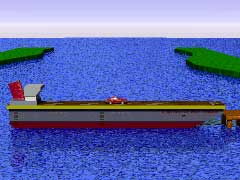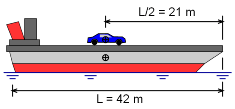Ch 4. Particle Momentum and Impulse Multimedia Engineering Dynamics Impulse & Momentum Consv. Linear Momentum Impact AngularMomentum MassFlow
 Chapter - Particle - 1. General Motion 2. Force & Accel. 3. Energy 4. Momentum - Rigid Body - 5. General Motion 6. Force & Accel. 7. Energy 8. Momentum 9. 3-D Motion 10. Vibrations Appendix Basic Math Units Basic Equations Sections Search eBooks Dynamics Fluids Math Mechanics Statics Thermodynamics Author(s): Kurt Gramoll ©Kurt GramollDYNAMICS - CASE STUDY IntroductionProblem Graphic A stunt man tries to jump a car from a floating barge to a dock. As the car accelerates forward, the barge pulls away from the dock. Will the car land on the dock? What is known: Both the car and the barge are at rest when the car starts to move. The mass of the car is mC = 500 kg. The mass of the barge is mB = 2500 kg. When the car reaches the end of the barge, the barge has a velocity of 0.885 m/s relative to the dock, moving away from the dock. The horizontal forces exerted on the barge by the water can be neglected.Problem Diagram Question When the car reaches the end of the barge, what are the velocity and position of the car relative to the dock? Approach When the car reaches the end of the barge, the momentum of the barge can be determined from its mass and velocity. The total linear momentum of the car and the barge is conserved in the horizontal direction. Use the conservation of linear momentum and the momentum of the barge to determine the momentum of the car when it reaches the end of the barge.

Practice Homework and Test problems now available in the 'Eng Dynamics' mobile app
Includes over 400 problems with complete detailed solutions.
Available now at the Google Play Store and Apple App Store.